# Maharashtra Board Class 5 Maths Solutions Chapter 4 Multiplication and Division Problem Set 15

Balbharti Maharashtra Board Class 5 Maths Solutions Chapter 4 Multiplication and Division Problem Set 15 Textbook Exercise Important Questions and Answers.

## Maharashtra State Board Class 5 Maths Solutions Chapter 4 Multiplication and Division Problem Set 15

Question 1.
Solve the following and write the quotient and remainder.
(1) 1284 ÷ 32
Solution :Quotient = 40
Remainder = 4

(2) 5586 ÷ 87
Solution :Quotient = 64
Remainder =18

(3) 1207 ÷ 27
Solution: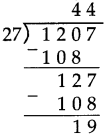Quotient = 44
Remainder =19

(4) 8543 ÷ 41
Solution :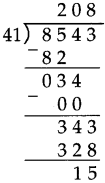Quotient = 208
Remainder =15

(5) 2304 ÷ 43
Solution:Quotient = 53
Remainder = 25

(6) 56,741 ÷ 26
Solution:Quotient =2182
Remainder = 9

Question 2.
How many hours will it take to travel 336 km at a speed of 48 km per hour?
Solution:
Time = Distance ÷ SpeedAnswer:
It will take 7 hours.

Question 3.
Girija needed 35 cartons to pack 1400 books. There are an equal number of books in every carton. How many books did she pack into each carton?
Solution:
No. of cartons x No. of books in each carton = Total no. of books 35 x No. of books in each carton = 1400 No. of books in each carton = 1400 35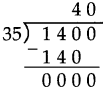Answer:
She packs 40 books in each carton.

Question 4.
The contribution for a picnic was 65 rupees each. Altogether, 2925 rupees were collected. How many had paid for the picnic?
Solution:Answer:
45 persons paid for the picnic.

Question 5.
Which number, on being multiplied by 56, gives a product of 9688?
Solution:Answer:
173

Question 6.
If 48 sheets are required for making one notebook, how many notebooks at the most will 5880 sheets make and how many sheets will be left over?
Solution:Answer:
122 notebooks can be made and 24 sheets left over.

Question 7.
What will the quotient be when the smallest five-digit number is divided by the smallest four-digit number?
Solution:
Smallest five-digit number is 10,000 and smallest four-digit number is 1,000.
So, 10000 ÷ 1000 = 10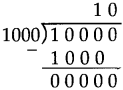Answer:
Quotient = 10

Mixed examples

A farmer brought 140 trays of chilli seedlings. Each tray had 24 seedlings. He planted all the seedlings in his field, putting 32 in a row. How many rows of chillies did he plant?

Let us find out the total number of seedlings when there were 24 seedlings in each of the 140 trays. We shall multiply 140 and 24.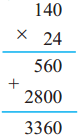Total number of seedlings 3,360.
To find out how many rows were planted with 32 seedlings in each row, we shall divide 3,360 by 32.
The quotient is 105.
Therefore, the number of rows is 105.
Carry out the multiplication of 105 × 32 and verify your answer.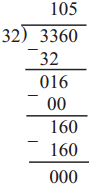Multiplication and Division Problem Set 15 Additional Important Questions and Answers

Solve the following and write the quotient and remainder.

(1) 9148 ÷ 37
Solution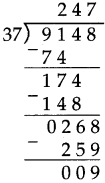Quotient = 247
Remainder = 9

(2) 1175 ÷ 15
Solution :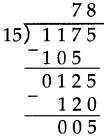Quotient =78
Remainder = 5

Solve the following word problems:

(1) If 45 kg of sugar cost 1305 rupees, what is the rate of sugar per kg?
Solution: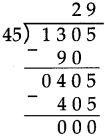Answer:
The rate per kg of sugar is 29 rupees.

(2) 17 people spent ₹ 83,475. How much did each person spend and what is the amount left?
Solution: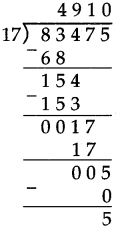Answer:
Each person spent ₹ 4,910 and the amount left is ₹ 5

Scroll to Top